## Tip: Quickly round the numbers in selected cells

Save 5 minutes a day by having ASAP Utilities quickly round the numbers for you.

## "Easily apply rounding to all numbers in your selection"

It is a common practice in Excel to format decimal places to get the desired rounding of numbers. But the problem with this approach is that it can display totals that don't appear to add up correctly.
For example, in the below screenshot it shows 2+2+2=7. That is because the underlying numbers are actually 2.44+2.46+1.42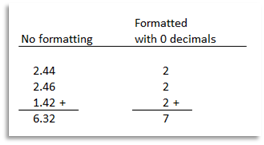The solution here is to use Excel's rounding functions.

A common approach is to use an extra 'helper' column to show the rounded results by using the =ROUND() function.
However, with ASAP Utilities it is also easy to round the number 'in place', in the cells themselves.

You can use one of the following tools to quickly round the numbers:

1. ASAP Utilities » Text » Insert before and/or after each cell in your selection...
This utility adds a value in front of and/or at the end of the content of each cell in your selection.
You can use this to wrap a formula around the contents of the cells.
The benefit of this is that you can still see the actual numbers.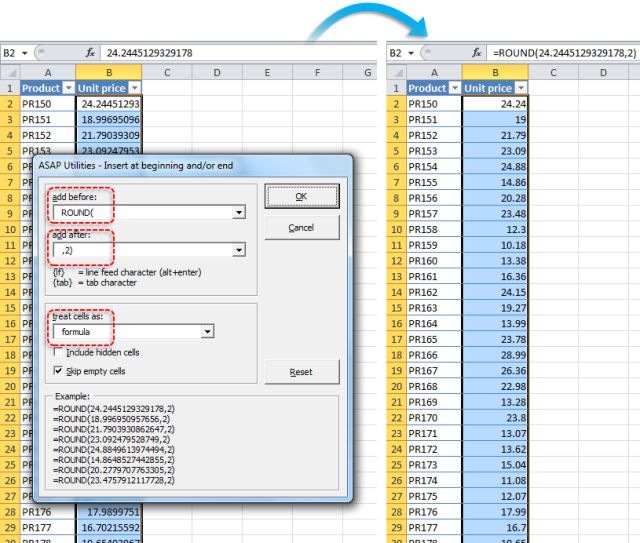2. ASAP Utilities » Numbers & Dates » Round numbers (not formatting, but changing)...
Instead of rounding the number by using cell formatting this tool will actually replace the value in the cells with the rounded value.Round the selected numbers to 2 decimals: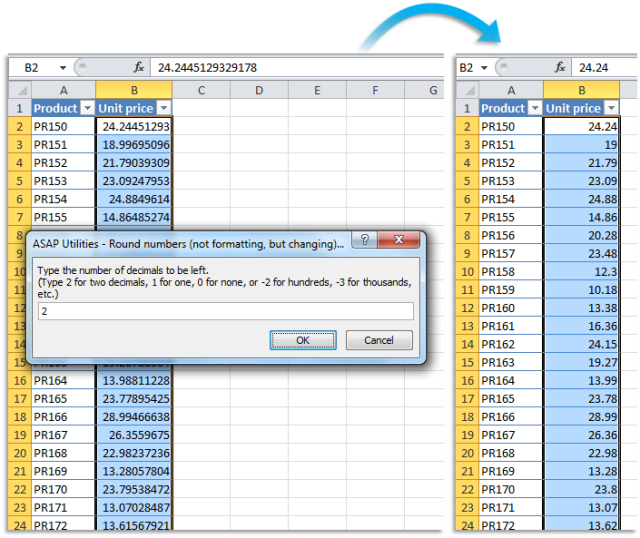Round the selected numbers to-the-nearest integer (zero decimal places):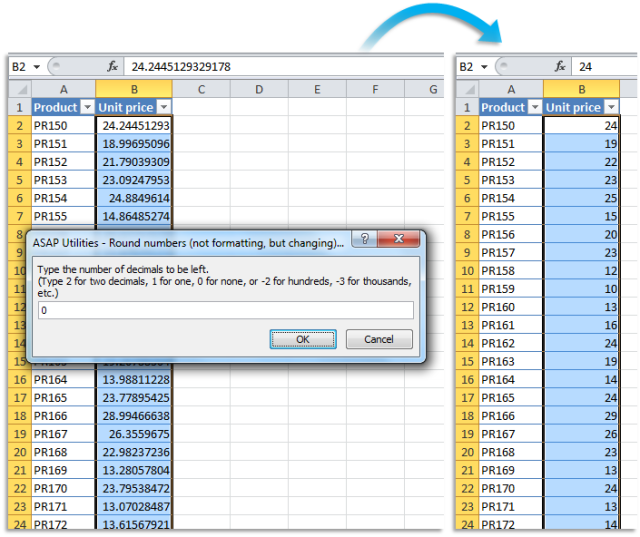Round the selected numbers to the nearest multiple of 100: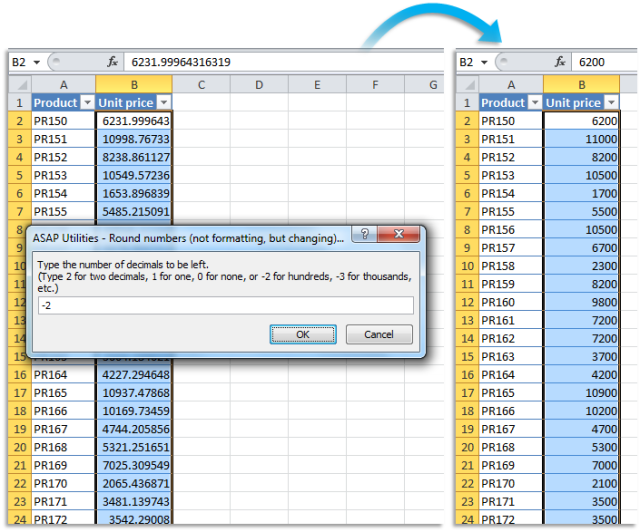## Do you recognize any of these situations?

• How do I apply a rounding formula to cells with different formulas easily?
I have a large spreadsheet that has different formulas in various cells. I have figured out how to round to two decimals as I wanted, but I need to apply that formula to all the other cells that have various formulas, without manually doing it for each cell.
• I have the amounts in column A. I know how to use the ROUND() function in B to get rounded result. However, is there a way to effectively format A1 to round the result of the formula it contains so I'm making use of one cell instead of two?
I can't just use the cell-formatting because formatting to two decimals just rounds the number for viewing; underlying number remains the same. The reason I need this is because I have a column of numbers which, when summed, have a result which appears to be different (usually just a cent) from the sum of the numbers as they are displayed.
Want to get rid of this 'rounding error' by actually rounding the numbers before summing them and if possible without an extra 'helper' column.
• I need some help with a spreadsheet I created to show me the net cost and vat of supplies.
The trouble is that totals are out by .01 dollar. After a lot of searching I found that by setting 'Set Precision as displayed' the totals were correct but I received a warning that data will permanently lose accuracy. So I'm not sure when should I be using this option and is this the correct approach to solve this rounding issue?

Just select the cells and use the following tool:
ASAP Utilities » Text » Insert before and/or after each cell in your selection...
and wrap around the needed function.
or use
ASAP Utilities » Numbers & Dates » Round numbers (not formatting, but changing)...

This is often easier than manually editing the formulas and it reduces the need for helper columns.
If you want to use a macro we've shared to do this.

### How much time will it save?

It's guaranteed that you'll save yourself time and effort by using this tool. However, the actual time saved depends on how much you use Excel, the amount of data you are working with and how often you use this particular tool.
You can easily see how much time ASAP Utilities has saved you so far.